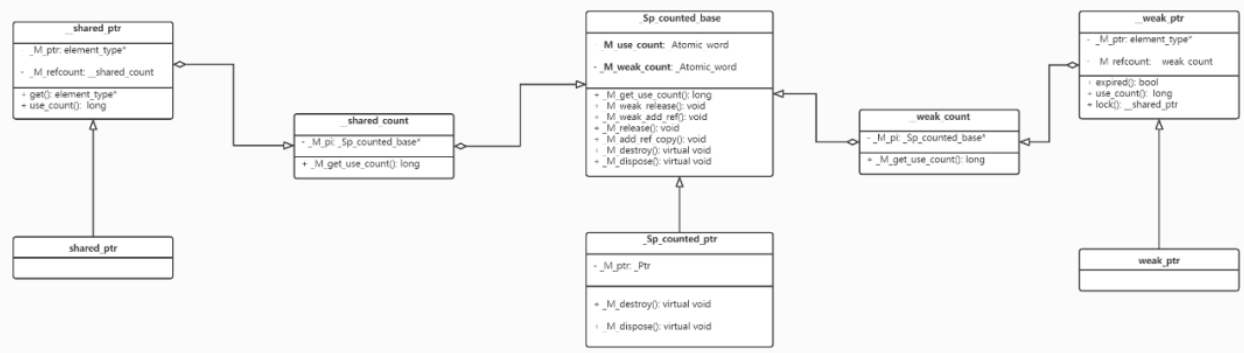# 源码分析 shared_ptr 实现

0收藏

• unique_ptr
• shared_ptr
• weak_ptr
关于智能指针使用以及区别可以自行查找资料，这里主要介绍智能指针的实现原理。

shared_ptr的实现_M_weak_count表示管理对象的引用计数，引用管理对象也是一个内存指针，这块指针是初始化第一个shared_ptr时new出来的，到最后也需要delete，所以使用_M_weak_count来控制管理对象什么时候析构，我们平时用到的weak_ptr内部其实持有的就是这个管理对象的指针，当weak_ptr拷贝时，管理对象的引用计数_M_weak_count就会增加，当_M_weak_count为0时，管理对象_M_pi就会析构且释放内存。

_M_use_count是如何加减的

_M_use_count表示托管对象的引用计数，即当shared_ptr拷贝时会增加，当shared_ptr析构时会减少，看代码：

``````template <typename _Yp>
__shared_ptr(const __shared_ptr<_Yp, _Lp>& __r,
element_type* __p) noexcept
: _M_ptr(__p), _M_refcount(__r._M_refcount)  // never throws
{
}

__shared_count(const __shared_count& __r) noexcept : _M_pi(__r._M_pi)
{
}

template <>
{
++_M_use_count;
}``````

``````template <typename _Yp>
_Assignable<const shared_ptr<_Yp>&> operator=(
const shared_ptr<_Yp>& __r) noexcept
{
this->__shared_ptr<_Tp>::operator=(__r);
return *this;
}

template <typename _Yp>
_Assignable<_Yp> operator=(const __shared_ptr<_Yp, _Lp>& __r) noexcept
{
_M_ptr = __r._M_ptr;
_M_refcount = __r._M_refcount;  // __shared_count::op= doesn't throw
return *this;
}

__shared_count& operator=(const __shared_count& __r) noexcept
{
_Sp_counted_base<_Lp>* __tmp = __r._M_pi;
if (__tmp != _M_pi) {
if (_M_pi != 0) _M_pi->_M_release();
_M_pi = __tmp;
}
return *this;
}``````

_M_use_count是如何减为0的，可以猜想到shared_ptr析构时会调用__shared_count的析构函数，

``````~__shared_count() noexcept
{
if (_M_pi != nullptr) _M_pi->_M_release();
}

template <>
inline void _Sp_counted_base<_S_single>::_M_release() noexcept
{
if (--_M_use_count == 0) {
_M_dispose();
if (--_M_weak_count == 0) _M_destroy();
}
}

virtual void _M_dispose() noexcept { delete _M_ptr; }``````

_M_weak_count是如何加减的

``_Sp_counted_base() noexcept : _M_use_count(1), _M_weak_count(1) {}``

``````__weak_count(const __weak_count& __r) noexcept : _M_pi(__r._M_pi)
{
}

template <>
{
++_M_weak_count;
}

~__weak_count() noexcept
{
if (_M_pi != nullptr) _M_pi->_M_weak_release();
}

template <>
inline void _Sp_counted_base<_S_single>::_M_weak_release() noexcept
{
if (--_M_weak_count == 0) _M_destroy();
}

virtual void _M_destroy() noexcept { delete this; }``````

weak_ptr的expired()和lock()做了什么

``````bool expired() const noexcept
{
return _M_refcount._M_get_use_count() == 0;
}``````

weak_ptr的expired()函数只是看了托管对象的引用计数是否为0，为0返回true

``````__shared_ptr<_Tp, _Lp> lock() const noexcept
{
return __shared_ptr<element_type, _Lp>(*this, std::nothrow);
}

__shared_ptr(const __weak_ptr<_Tp, _Lp>& __r, std::nothrow_t)
: _M_refcount(__r._M_refcount, std::nothrow)
{
_M_ptr = _M_refcount._M_get_use_count() ? __r._M_ptr : nullptr;
}``````

weak_ptr的lock()函数是打算返回一个shared_ptr对象来延长托管对象的生命周期，这里返回后需要判断返回值是否为nullptr。

``shared_from_this()是如何实现的``

``````class enable_shared_from_this
{
shared_ptr<const _Tp> shared_from_this() const
{
return shared_ptr<const _Tp>(this->_M_weak_this);
}

mutable weak_ptr<_Tp> _M_weak_this;
};``````

shared_ptr内部使用__shared_count中的_Sp_counted_base对象来控制托管指针，_Sp_counted_base内部有_M_use_count和_M_weak_count，_M_use_count表示托管指针的引用计数，_M_weak_count表示_Sp_counted_base的引用计数，_M_use_count为0时候释放托管指针指向的内存，_M_weak_count为0时释放_Sp_counted_base指向的内存，这里_Sp_counted_base的生命线一般不会短于shared_ptr的生命线。

1帖子
视频
声望
粉丝
社区精华内容